0%
Note - These are my notes on DeepLearning Specialization Part: Logistic Regression with a Neural Network mindset || (Course- 1 Week - 2) || Neural Networks and Deep Learning (Week 2)

# Logistic Regression with a Neural Network mindset

Welcome to your first (required) programming assignment! You will build a logistic regression classifier to recognize cats. This assignment will step you through how to do this with a Neural Network mindset, and so will also hone your intuitions about deep learning.

Instructions:

• Do not use loops (for/while) in your code, unless the instructions explicitly ask you to do so.

You will learn to:

• Build the general architecture of a learning algorithm, including:
• Initializing parameters
• Calculating the cost function and its gradient
• Using an optimization algorithm (gradient descent)
• Gather all three functions above into a main model function, in the right order.

## 1 - Packages

First, let’s run the cell below to import all the packages that you will need during this assignment.

• numpy is the fundamental package for scientific computing with Python.
• h5py is a common package to interact with a dataset that is stored on an H5 file.
• matplotlib is a famous library to plot graphs in Python.
• PIL and scipy are used here to test your model with your own picture at the end.

## 2 - Overview of the Problem set

Problem Statement: You are given a dataset (“data.h5”) containing:
- a training set of m_train images labeled as cat (y=1) or non-cat (y=0)
- a test set of m_test images labeled as cat or non-cat
- each image is of shape (num_px, num_px, 3) where 3 is for the 3 channels (RGB). Thus, each image is square (height = num_px) and (width = num_px).

You will build a simple image-recognition algorithm that can correctly classify pictures as cat or non-cat.

Let’s get more familiar with the dataset. Load the data by running the following code.

We added “_orig” at the end of image datasets (train and test) because we are going to preprocess them. After preprocessing, we will end up with train_set_x and test_set_x (the labels train_set_y and test_set_y don’t need any preprocessing).

Each line of your train_set_x_orig and test_set_x_orig is an array representing an image. You can visualize an example by running the following code. Feel free also to change the index value and re-run to see other images.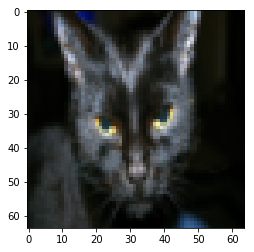Many software bugs in deep learning come from having matrix/vector dimensions that don’t fit. If you can keep your matrix/vector dimensions straight you will go a long way toward eliminating many bugs.

Exercise: Find the values for:
- m_train (number of training examples)
- m_test (number of test examples)
- num_px (= height = width of a training image)
Remember that train_set_x_orig is a numpy-array of shape (m_train, num_px, num_px, 3). For instance, you can access m_train by writing train_set_x_orig.shape.

Number of training examples: m_train = 209
Number of testing examples: m_test = 50
Height/Width of each image: num_px = 64
Each image is of size: (64, 64, 3)
train_set_x shape: (209, 64, 64, 3)
train_set_y shape: (1, 209)
test_set_x shape: (50, 64, 64, 3)
test_set_y shape: (1, 50)


Expected Output for m_train, m_test and num_px:

 **m_train** 209 **m_test** 50 **num_px** 64

For convenience, you should now reshape images of shape (num_px, num_px, 3) in a numpy-array of shape (num_px $$num_px$$ 3, 1). After this, our training (and test) dataset is a numpy-array where each column represents a flattened image. There should be m_train (respectively m_test) columns.

Exercise: Reshape the training and test data sets so that images of size (num_px, num_px, 3) are flattened into single vectors of shape (num_px $$num_px$$ 3, 1).

A trick when you want to flatten a matrix X of shape (a,b,c,d) to a matrix X_flatten of shape (b$$c$$d, a) is to use:

train_set_x_flatten shape: (12288, 209)
train_set_y shape: (1, 209)
test_set_x_flatten shape: (12288, 50)
test_set_y shape: (1, 50)
sanity check after reshaping: [17 31 56 22 33]


Expected Output:

 **train_set_x_flatten shape** (12288, 209) **train_set_y shape** (1, 209) **test_set_x_flatten shape** (12288, 50) **test_set_y shape** (1, 50) **sanity check after reshaping** [17 31 56 22 33]

To represent color images, the red, green and blue channels (RGB) must be specified for each pixel, and so the pixel value is actually a vector of three numbers ranging from 0 to 255.

One common preprocessing step in machine learning is to center and standardize your dataset, meaning that you substract the mean of the whole numpy array from each example, and then divide each example by the standard deviation of the whole numpy array. But for picture datasets, it is simpler and more convenient and works almost as well to just divide every row of the dataset by 255 (the maximum value of a pixel channel).

Let’s standardize our dataset.

Out - 12288

What you need to remember:

Common steps for pre-processing a new dataset are:

• Figure out the dimensions and shapes of the problem (m_train, m_test, num_px, …)
• Reshape the datasets such that each example is now a vector of size (num_px * num_px * 3, 1)
• “Standardize” the data

## 3 - General Architecture of the learning algorithm

It’s time to design a simple algorithm to distinguish cat images from non-cat images.

Mathematical expression of the algorithm:

For one example $x^{(i)}$:
$$z^{(i)} = w^T x^{(i)} + b \tag{1}$$
$$\hat{y}^{(i)} = a^{(i)} = sigmoid(z^{(i)})\tag{2}$$
$$\mathcal{L}(a^{(i)}, y^{(i)}) = - y^{(i)} \log(a^{(i)}) - (1-y^{(i)} ) *$$

Key steps:
In this exercise, you will carry out the following steps:
- Initialize the parameters of the model
- Learn the parameters for the model by minimizing the cost
- Use the learned parameters to make predictions (on the test set)
- Analyse the results and conclude

## 4 - Building the parts of our algorithm

The main steps for building a Neural Network are:

1. Define the model structure (such as number of input features)
2. Initialize the model’s parameters
3. Loop:
• Calculate current loss (forward propagation)
• Calculate current gradient (backward propagation)

You often build 1-3 separately and integrate them into one function we call model().

### 4.1 - Helper functions

Exercise: Using your code from “Python Basics”, implement sigmoid(). As you’ve seen in the figure above, you need to compute $sigmoid( w^T x + b) = \frac{1}{1 + e^{-(w^T x + b)}}$ to make predictions. Use np.exp().

sigmoid([0, 2]) = [0.5        0.88079708]


Expected Output:

 **sigmoid([0, 2])** [ 0.5 0.88079708]

### 4.2 - Initializing parameters

Exercise: Implement parameter initialization in the cell below. You have to initialize w as a vector of zeros. If you don’t know what numpy function to use, look up np.zeros() in the Numpy library’s documentation.

w = [[0.]
[0.]]
b = 0


Expected Output:

 ** w ** [[ 0.] [ 0.]] ** b ** 0

For image inputs, w will be of shape (num_px $\times$ num_px $\times$ 3, 1).

### 4.3 - Forward and Backward propagation

Now that your parameters are initialized, you can do the “forward” and “backward” propagation steps for learning the parameters.

Exercise: Implement a function propagate() that computes the cost function and its gradient.

Hints:

Forward Propagation:

• You get X
• You compute $A = \sigma(w^T X + b) = (a^{(0)}, a^{(1)}, …, a^{(m-1)}, a^{(m)})$
• You calculate the cost function: $J = -\frac{1}{m}\sum_{i=1}^{m}y^{(i)}\log(a^{(i)})+(1-y^{(i)})\log(1-a^{(i)})$

Here are the two formulas you will be using:

$$\frac{\partial J}{\partial w} = \frac{1}{m}X(A-Y)^T\tag{7}$$
$$\frac{\partial J}{\partial b} = \frac{1}{m} \sum_{i=1}^m (a^{(i)}-y^{(i)})\tag{8}$$

dw = [[0.99993216]
[1.99980262]]
db = [0.49993523]
cost = 6.000064773192205


Expected Output:

 ** dw ** [[ 0.99993216] [ 1.99980262]] ** db ** 0.499935230625 ** cost ** 6.000064773192205

### d) Optimization

• You have initialized your parameters.
• You are also able to compute a cost function and its gradient.
• Now, you want to update the parameters using gradient descent.

Exercise: Write down the optimization function. The goal is to learn $w$ and $b$ by minimizing the cost function $J$. For a parameter $\theta$, the update rule is $\theta = \theta - \alpha \text{ } d\theta$, where $\alpha$ is the learning rate.

w = [[0.1124579 ]
[0.23106775]]
b = [1.55930492]
dw = [[0.90158428]
[1.76250842]]
db = [0.43046207]


Expected Output:

<tr>
<td> **b** </td>
<td> 1.55930492484 </td>
</tr>
<tr>
<td> **dw** </td>
<td> [[ 0.90158428]


[ 1.76250842]]

 **w** [[ 0.1124579 ] [ 0.23106775]] db 0.430462071679

Exercise: The previous function will output the learned w and b. We are able to use w and b to predict the labels for a dataset X. Implement the predict() function. There is two steps to computing predictions:

1. Calculate $\hat{Y} = A = \sigma(w^T X + b)$

2. Convert the entries of a into 0 (if activation <= 0.5) or 1 (if activation > 0.5), stores the predictions in a vector Y_prediction. If you wish, you can use an if/else statement in a for loop (though there is also a way to vectorize this).

[0.99987661 0.99999386]
predictions = [[1. 1.]]


Expected Output:

 **predictions** [[ 1. 1.]]

What to remember

You’ve implemented several functions that:

• Initialize (w,b)
• Optimize the loss iteratively to learn parameters (w,b):
• computing the cost and its gradient
• updating the parameters using gradient descent
• Use the learned (w,b) to predict the labels for a given set of examples

## 5 - Merge all functions into a model

You will now see how the overall model is structured by putting together all the building blocks (functions implemented in the previous parts) together, in the right order.

Exercise: Implement the model function. Use the following notation:
- Y_prediction for your predictions on the test set
- Y_prediction_train for your predictions on the train set
- w, costs, grads for the outputs of optimize()

Run the following cell to train your model.

Cost after iteration 0: 0.693147
Cost after iteration 100: 0.584508
Cost after iteration 200: 0.466949
Cost after iteration 300: 0.376007
Cost after iteration 400: 0.331463
Cost after iteration 500: 0.303273
Cost after iteration 600: 0.279880
Cost after iteration 700: 0.260042
Cost after iteration 800: 0.242941
Cost after iteration 900: 0.228004
Cost after iteration 1000: 0.214820
Cost after iteration 1100: 0.203078
Cost after iteration 1200: 0.192544
Cost after iteration 1300: 0.183033
Cost after iteration 1400: 0.174399
Cost after iteration 1500: 0.166521
Cost after iteration 1600: 0.159305
Cost after iteration 1700: 0.152667
Cost after iteration 1800: 0.146542
Cost after iteration 1900: 0.140872
[0.94366988 0.86095311 0.88896715 0.93630641 0.74075403 0.52849619
0.03094677 0.85707681 0.88457925 0.67279696 0.26601085 0.4823794
0.74741157 0.78575729 0.00978911 0.9203284  0.02453695 0.84884703
0.2050248  0.03703224 0.92931392 0.11930532 0.01411064 0.7832698
0.58188015 0.66897565 0.75119007 0.01323558 0.03402649 0.99735115
0.21031727 0.78123225 0.6815842  0.46647604 0.66323375 0.03424828
0.08031627 0.76570656 0.34760863 0.06177743 0.6987531  0.4106426
0.6648871  0.02776868 0.93053125 0.46395717 0.23971605 0.9771735
0.66202407 0.10482388]
[1.96533335e-01 8.97519936e-02 8.90887727e-01 2.05354859e-04
4.10043201e-02 1.13855541e-01 3.58425358e-02 9.20256043e-01
8.11815498e-02 5.09505652e-02 1.43687735e-01 7.77661312e-01
2.37002682e-01 9.26822611e-01 7.20256211e-01 4.54525029e-02
2.88164240e-02 4.96209946e-02 9.53642451e-02 9.27127783e-01
1.46871713e-02 4.42749993e-02 1.99658284e-01 5.10794145e-02
8.71854257e-01 8.54873232e-01 4.43988460e-02 8.41877286e-01
5.57178266e-02 7.39175253e-01 8.73390575e-02 7.61255429e-02
2.01282223e-01 2.02159519e-01 7.95065561e-02 3.69885691e-02
1.14655638e-02 5.90397260e-02 8.36880946e-01 3.33057415e-01
1.98548242e-02 4.46965063e-01 8.23737950e-01 4.13465923e-02
4.61512591e-02 1.21739845e-01 9.76716144e-02 8.07086225e-01
8.93389416e-03 3.73249849e-02 7.53711249e-01 2.47934596e-01
1.47013078e-01 3.93089594e-01 9.02530607e-01 3.94290174e-03
9.38300399e-01 8.14429890e-01 5.51201724e-02 9.56820776e-01
8.35826040e-01 7.75371183e-01 4.97406386e-02 5.05302748e-02
1.68276426e-01 7.39795683e-02 4.23114248e-02 1.80374321e-01
7.36839673e-01 2.36170561e-02 4.78407244e-02 9.72682719e-01
8.87430447e-02 1.40500115e-01 7.39006094e-02 5.87414480e-01
8.55122639e-04 3.51320419e-02 7.21341360e-02 1.59367000e-01
9.18793718e-02 2.76678199e-03 2.16954763e-02 8.75788002e-01
7.48905473e-01 2.61224310e-02 1.31264831e-01 5.58549892e-02
7.96470422e-01 4.31114360e-02 2.46081640e-01 9.28094796e-02
5.13207713e-01 9.23532733e-01 9.11010943e-01 1.56664277e-01
1.40529680e-01 8.72871654e-01 6.33390909e-02 2.04276699e-01
1.50378528e-01 5.42005811e-02 7.16869008e-01 8.93930822e-02
9.68748123e-01 1.16897229e-01 9.65813244e-01 7.63463753e-01
8.45184245e-01 7.94804824e-01 8.77046596e-01 8.92528474e-01
2.33698759e-02 1.08088606e-01 9.41045938e-02 5.06133571e-02
6.14255764e-02 8.74814031e-01 7.14021606e-03 1.49573407e-01
1.38752636e-02 5.75050572e-01 4.74218632e-02 2.67728414e-04
8.16437270e-01 5.25431990e-03 8.27320337e-01 1.63520986e-01
9.19597717e-01 9.11124533e-01 2.96731271e-01 1.37316359e-01
7.56632692e-02 9.51896490e-01 7.13340131e-01 5.62771203e-01
8.46803645e-01 8.81283783e-01 5.80214923e-03 3.24191787e-02
3.66569448e-02 4.24241240e-02 9.02746461e-01 6.95602248e-03
7.28528692e-01 8.04734016e-01 8.48847026e-01 1.97286016e-01
8.73972266e-01 8.56810568e-01 4.60108117e-01 9.98074787e-02
2.67726747e-02 9.16713593e-01 5.70477051e-02 2.34413956e-01
9.17441504e-01 1.43642340e-02 1.48384241e-02 4.18971050e-02
4.81257763e-03 6.74987512e-02 7.96958661e-01 7.94548221e-02
8.88055227e-01 1.63703299e-02 9.64896262e-01 4.74597209e-02
3.78354422e-02 6.75950812e-01 7.60983832e-01 8.91154251e-01
2.15482871e-01 1.80695199e-02 9.46591763e-01 7.71101522e-01
4.14565207e-02 8.02916154e-01 8.02541805e-02 6.89037478e-01
4.92103989e-02 4.87010785e-02 1.89987579e-02 3.71043577e-02
1.73595068e-03 7.71575747e-01 4.06433366e-02 6.60606392e-02
8.34508562e-01 2.27408842e-02 6.17839573e-02 4.56149270e-02
7.64947622e-01 6.19347921e-02 3.55887869e-03 1.03103435e-01
3.83745905e-01 8.77909931e-01 7.72818586e-02 1.79082665e-02
8.09911232e-01 2.02130387e-02 1.89353139e-02 1.83142729e-02
1.94041166e-01 2.01151983e-01 8.48224028e-02 1.61929290e-01
1.82858623e-01]
train accuracy: 99.04306220095694 %
test accuracy: 70.0 %


Expected Output:

<tr>
<td> **Train Accuracy**  </td>
<td> 99.04306220095694 % </td>
</tr>

<tr>
<td>**Test Accuracy** </td>
<td> 70.0 % </td>
</tr>


Comment: Training accuracy is close to 100%. This is a good sanity check: your model is working and has high enough capacity to fit the training data. Test error is 68%. It is actually not bad for this simple model, given the small dataset we used and that logistic regression is a linear classifier. But no worries, you’ll build an even better classifier next week!

Also, you see that the model is clearly overfitting the training data. Later in this specialization you will learn how to reduce overfitting, for example by using regularization. Using the code below (and changing the index variable) you can look at predictions on pictures of the test set.

y = 1, you predicted that it is a "cat" picture.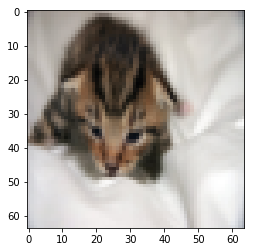Let’s also plot the cost function and the gradients.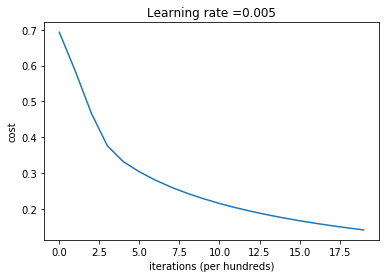Interpretation:
You can see the cost decreasing. It shows that the parameters are being learned. However, you see that you could train the model even more on the training set. Try to increase the number of iterations in the cell above and rerun the cells. You might see that the training set accuracy goes up, but the test set accuracy goes down. This is called overfitting.

## 6 - Further analysis (optional/ungraded exercise)

Congratulations on building your first image classification model. Let’s analyze it further, and examine possible choices for the learning rate $\alpha$.

#### Choice of learning rate

Reminder:
In order for Gradient Descent to work you must choose the learning rate wisely. The learning rate $\alpha$ determines how rapidly we update the parameters. If the learning rate is too large we may “overshoot” the optimal value. Similarly, if it is too small we will need too many iterations to converge to the best values. That’s why it is crucial to use a well-tuned learning rate.

Let’s compare the learning curve of our model with several choices of learning rates. Run the cell below. This should take about 1 minute. Feel free also to try different values than the three we have initialized the learning_rates variable to contain, and see what happens.

learning rate is: 0.01
[0.97125943 0.9155338  0.92079132 0.96358044 0.78924234 0.60411297
0.01179527 0.89814048 0.91522859 0.70264065 0.19380387 0.49537355
0.7927164  0.85423431 0.00298587 0.96199699 0.01234735 0.9107653
0.13661137 0.01424336 0.96894735 0.1033746  0.00579297 0.86081326
0.53811196 0.64950178 0.83272843 0.00426307 0.0131452  0.99947804
0.11468372 0.82182442 0.69611733 0.4991522  0.67231401 0.01728165
0.04136099 0.80069693 0.26832359 0.03958566 0.74731239 0.32116434
0.71871197 0.01205725 0.96879962 0.62310364 0.17737126 0.98960523
0.74697265 0.07284605]
[1.47839654e-01 5.78008187e-02 9.42385025e-01 4.14849240e-05
2.27209941e-02 7.29254668e-02 2.23704494e-02 9.49717864e-01
5.41724296e-02 2.92729895e-02 6.82412300e-02 8.33370210e-01
1.71420615e-01 9.66879883e-01 8.11537151e-01 2.44343483e-02
7.87634098e-03 2.64027272e-02 5.60720049e-02 9.53130353e-01
5.30865324e-03 3.11020745e-02 1.43606493e-01 1.92650473e-02
9.30132798e-01 8.95291211e-01 2.72790550e-02 9.01480142e-01
2.73987904e-02 8.09041583e-01 6.64266068e-02 5.00479730e-02
1.29245158e-01 1.40274640e-01 6.48179131e-02 1.35261338e-02
4.77693620e-03 2.65922710e-02 8.89771230e-01 2.64826222e-01
1.22921585e-02 6.03229153e-01 8.81822076e-01 1.35079742e-02
2.49595285e-02 6.88961126e-02 5.86046930e-02 8.68932415e-01
5.14520331e-03 1.21099845e-02 8.23403970e-01 1.70985647e-01
9.49977566e-02 3.04227660e-01 9.48091298e-01 8.09204729e-04
9.66640038e-01 8.78319466e-01 3.17284880e-02 9.76165700e-01
8.81584697e-01 8.48145722e-01 2.70795160e-02 2.28390397e-02
1.05295676e-01 4.45165292e-02 1.22858876e-02 1.35813814e-01
8.25867437e-01 9.21552651e-03 2.49353830e-02 9.88067070e-01
5.78381495e-02 8.57292849e-02 4.10128551e-02 5.70507956e-01
2.11603229e-04 1.52264723e-02 6.18390722e-02 1.39187810e-01
6.68993749e-02 4.14281785e-04 1.23347660e-02 9.24789062e-01
8.16880995e-01 9.29503655e-03 8.23770893e-02 2.75905821e-02
8.52215781e-01 2.36580782e-02 1.75344552e-01 6.15499363e-02
6.58017000e-01 9.54697511e-01 9.62775471e-01 1.05372217e-01
9.37239413e-02 9.29062265e-01 2.68654456e-02 1.44668290e-01
9.15662947e-02 2.89260930e-02 8.02603133e-01 6.11847790e-02
9.87937140e-01 5.84677169e-02 9.87171184e-01 8.37167548e-01
8.94717386e-01 8.58260204e-01 9.36232298e-01 9.33067878e-01
8.77279900e-03 5.88387682e-02 5.09517612e-02 2.40626781e-02
3.87480256e-02 9.35343373e-01 2.35202639e-03 8.83972091e-02
4.49639004e-03 6.64404296e-01 1.76677024e-02 2.75426440e-05
8.71728805e-01 2.43292078e-03 8.92351131e-01 9.50411299e-02
9.66495010e-01 9.27285472e-01 2.66413779e-01 8.70883114e-02
5.40743542e-02 9.75155426e-01 8.02323751e-01 6.92965782e-01
9.06287458e-01 9.39900204e-01 1.64790714e-03 1.91364329e-02
1.66925680e-02 1.46846281e-02 9.39237709e-01 2.57925925e-03
8.19134439e-01 8.54311895e-01 9.10765301e-01 1.20452016e-01
9.10603560e-01 9.11977137e-01 3.72174950e-01 6.13527932e-02
1.30882743e-02 9.55225821e-01 4.30680816e-02 1.37970158e-01
9.60868956e-01 8.67705030e-03 5.95741909e-03 2.19466774e-02
1.78308409e-03 2.57658927e-02 8.63787547e-01 3.44218954e-02
9.34152347e-01 9.35483274e-03 9.90908018e-01 1.17832722e-02
2.67756870e-02 7.74546160e-01 8.43831858e-01 9.38847463e-01
1.48599256e-01 4.17198956e-03 9.81043189e-01 8.22764984e-01
1.92120393e-02 8.58870443e-01 5.37478573e-02 7.84878423e-01
3.56080493e-02 2.80545014e-02 1.09777935e-02 1.30396160e-02
3.81067987e-04 8.51025984e-01 2.44476492e-02 4.57657708e-02
8.81871553e-01 1.06481927e-02 2.84032919e-02 1.96773463e-02
8.54577180e-01 3.01055581e-02 1.33843958e-03 7.04152762e-02
3.08344786e-01 9.25167630e-01 4.53183035e-02 9.31980521e-03
8.69872444e-01 4.61339718e-03 4.86286962e-03 7.32772398e-03
1.26009270e-01 1.46124056e-01 4.51019670e-02 1.45139959e-01
1.45971589e-01]
train accuracy: 99.52153110047847 %
test accuracy: 68.0 %

-------------------------------------------------------

learning rate is: 0.001
[0.74458179 0.63302701 0.70621076 0.7037801  0.5322598  0.43784581
0.1843739  0.71778574 0.73717649 0.59122536 0.39837511 0.44491784
0.63244572 0.53976962 0.09938522 0.7227688  0.12316033 0.58301417
0.28145733 0.16609522 0.61461919 0.14166416 0.0865388  0.4251847
0.67719513 0.61251308 0.46730808 0.11854922 0.21041046 0.8906756
0.42313203 0.56013238 0.60322016 0.37148913 0.57460259 0.11968291
0.24088599 0.65905854 0.4782032  0.14862075 0.4992436  0.61682528
0.4795275  0.16260336 0.70722369 0.23929218 0.36719514 0.87223907
0.45484261 0.19029187]
[0.34403391 0.18575705 0.63392388 0.00949352 0.185803   0.30979007
0.13544854 0.75931407 0.18856286 0.16653711 0.47903517 0.55094252
0.39894694 0.67613631 0.32941411 0.15120523 0.1515817  0.10868391
0.21533234 0.78261458 0.09236643 0.13102179 0.30209379 0.22018859
0.60467471 0.63089631 0.13786841 0.52162666 0.2229145  0.41807311
0.20928386 0.22354737 0.51863273 0.37446655 0.12619979 0.24763606
0.08217106 0.20570627 0.61668309 0.47341694 0.07578526 0.20272218
0.63694514 0.17332725 0.12774778 0.38987251 0.25716102 0.57589232
0.03660729 0.23627192 0.5058546  0.44851881 0.26882028 0.54506441
0.63427748 0.07593065 0.79389128 0.55848777 0.20399827 0.82950311
0.67551516 0.49340246 0.12825017 0.19483707 0.30405843 0.25239064
0.23849329 0.28306742 0.39562206 0.1338017  0.20953382 0.84559705
0.25983452 0.43347997 0.25869745 0.5275365  0.01851016 0.18226072
0.11686925 0.24360522 0.11457144 0.09711829 0.11403479 0.64158072
0.56492264 0.14249209 0.26621215 0.23562087 0.63347539 0.19718838
0.41665293 0.2560914  0.20511226 0.75854285 0.62700096 0.22437352
0.24158168 0.58986637 0.18250551 0.31168748 0.40230892 0.18766222
0.37363736 0.24954905 0.81540625 0.33905562 0.85287524 0.46460165
0.64873862 0.49476607 0.58689285 0.73160658 0.15974705 0.28192355
0.21969254 0.17213348 0.24140747 0.59506433 0.09843999 0.4664941
0.11789794 0.41615495 0.28828188 0.01549565 0.57657208 0.03491378
0.56433333 0.52342054 0.58263447 0.828261   0.33112864 0.30054751
0.13866344 0.7796039  0.49905825 0.23849455 0.65130553 0.54865883
0.07475945 0.15289783 0.17277205 0.21093974 0.77996081 0.05731401
0.43542011 0.62528802 0.58301417 0.39592429 0.62711359 0.62164606
0.52142034 0.2237536  0.11263677 0.69875451 0.13460421 0.59843604
0.62542932 0.06223702 0.08147044 0.16677973 0.0471795  0.29026597
0.53465382 0.37298272 0.67567251 0.08157721 0.80300364 0.36035876
0.12411481 0.3216639  0.50044148 0.71061923 0.38536321 0.15865892
0.73153708 0.60089581 0.19123039 0.60169201 0.21145324 0.35174627
0.1036799  0.15432715 0.08266478 0.1765554  0.0315303  0.4005418
0.12684962 0.13818573 0.69657105 0.10353719 0.15229696 0.170385
0.39005657 0.21251557 0.03788715 0.32853945 0.47339083 0.62631301
0.22739755 0.0902158  0.56782331 0.17507791 0.20252309 0.09500011
0.34504767 0.39618939 0.25822558 0.24550552 0.30677401]
train accuracy: 88.99521531100478 %
test accuracy: 64.0 %

-------------------------------------------------------

learning rate is: 0.0001
[0.45098635 0.48539489 0.40959087 0.44864257 0.32818894 0.43729766
0.28884626 0.46438078 0.45494399 0.45491705 0.36938309 0.41863679
0.45816519 0.5031755  0.2842568  0.45155065 0.30672371 0.37824086
0.26505548 0.27737934 0.40677576 0.28781555 0.24304775 0.38397796
0.50642581 0.47047843 0.35358916 0.31561491 0.39430714 0.4603235
0.37998879 0.3764821  0.32056264 0.38693085 0.40764828 0.23150119
0.311659   0.44981144 0.43152263 0.26276732 0.37785575 0.48883282
0.37790798 0.30969512 0.47842906 0.32857529 0.34457076 0.60547775
0.40733226 0.28828383]
[0.4225819  0.31692389 0.42964509 0.14896683 0.28783033 0.38652698
0.29492571 0.44991522 0.31988018 0.32391139 0.39318147 0.34804173
0.40099138 0.31694856 0.28102266 0.3231201  0.25486297 0.18485428
0.31900054 0.52941528 0.25568417 0.27297382 0.29762542 0.35834172
0.38912252 0.4552143  0.2555983  0.34830216 0.29078565 0.27432926
0.31094887 0.44330557 0.47172673 0.39765449 0.22386371 0.46108148
0.27055987 0.31333951 0.49901097 0.439851   0.23953174 0.29809115
0.42197081 0.28385499 0.2465556  0.40478121 0.35487343 0.45521241
0.1451398  0.37485678 0.36671611 0.37909623 0.30298036 0.40151709
0.40460677 0.24757226 0.50122617 0.38917296 0.3687779  0.50666786
0.52492017 0.37864634 0.24031899 0.30627306 0.35114005 0.37398054
0.43104844 0.31851314 0.37029232 0.29232461 0.37616632 0.52373453
0.32507684 0.48381803 0.39170698 0.38646363 0.17397111 0.31623794
0.24714356 0.35235176 0.17699762 0.33409149 0.3249697  0.49338319
0.413497   0.25575577 0.31724095 0.36456893 0.45018347 0.34698807
0.38500817 0.46053573 0.23091555 0.47268593 0.43291929 0.26828088
0.30258929 0.4164904  0.24453098 0.27111067 0.39272427 0.3043153
0.27984468 0.39085873 0.52409691 0.34966557 0.55950717 0.37739831
0.43697184 0.33557455 0.38439647 0.48607992 0.30731772 0.31028645
0.30037443 0.29400226 0.42714997 0.42909528 0.30432821 0.5227302
0.32144602 0.4066469  0.43683178 0.17768611 0.4354696  0.18662466
0.39558874 0.48819809 0.35425959 0.57279833 0.35098941 0.33429763
0.31014998 0.49175402 0.44154511 0.31203466 0.38776576 0.38352489
0.29611222 0.36104647 0.33824864 0.37198268 0.52831432 0.25732676
0.36743298 0.44902822 0.37824086 0.36790056 0.41246464 0.37833397
0.3418507  0.30119593 0.2592477  0.46753926 0.26777792 0.43134603
0.32491304 0.19700069 0.25937972 0.33143626 0.19820128 0.35468513
0.36334932 0.51823778 0.37235121 0.27650473 0.47271147 0.44760504
0.33240186 0.29967323 0.41157608 0.47817969 0.39048545 0.28309008
0.46350184 0.41099669 0.34508275 0.4323286  0.35065016 0.33976266
0.25459527 0.29233107 0.26976618 0.30004182 0.18212017 0.34254174
0.26213135 0.2674514  0.45817075 0.24356149 0.25227369 0.34588203
0.3783451  0.32762257 0.19831251 0.51451759 0.3792938  0.41417054
0.34795587 0.25521854 0.42313521 0.32557428 0.38342989 0.21943589
0.34909483 0.39399177 0.36128874 0.38042346 0.38929593]
train accuracy: 68.42105263157895 %
test accuracy: 36.0 %

-------------------------------------------------------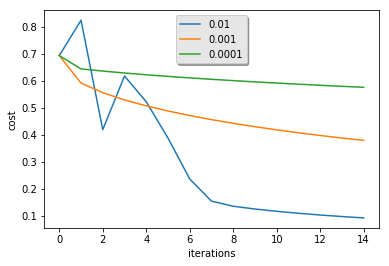Interpretation:

• Different learning rates give different costs and thus different predictions results.
• If the learning rate is too large (0.01), the cost may oscillate up and down. It may even diverge (though in this example, using 0.01 still eventually ends up at a good value for the cost).
• A lower cost doesn’t mean a better model. You have to check if there is possibly overfitting. It happens when the training accuracy is a lot higher than the test accuracy.
• In deep learning, we usually recommend that you:
• Choose the learning rate that better minimizes the cost function.

Congratulations on finishing this assignment. You can use your own image and see the output of your model. To do that:
1. Click on “File” in the upper bar of this notebook, then click “Open” to go on your Coursera Hub.
2. Add your image to this Jupyter Notebook’s directory, in the “images” folder
3. Change your image’s name in the following code
4. Run the code and check if the algorithm is right (1 = cat, 0 = non-cat)!

/Users/abanihi/opt/miniconda3/envs/pangeo/lib/python3.6/site-packages/skimage/transform/_warps.py:105: UserWarning: The default mode, 'constant', will be changed to 'reflect' in skimage 0.15.
warn("The default mode, 'constant', will be changed to 'reflect' in "
/Users/abanihi/opt/miniconda3/envs/pangeo/lib/python3.6/site-packages/skimage/transform/_warps.py:110: UserWarning: Anti-aliasing will be enabled by default in skimage 0.15 to avoid aliasing artifacts when down-sampling images.
warn("Anti-aliasing will be enabled by default in skimage 0.15 to "

[0.30930831]
y = 0.0, your algorithm predicts a "non-cat" picture.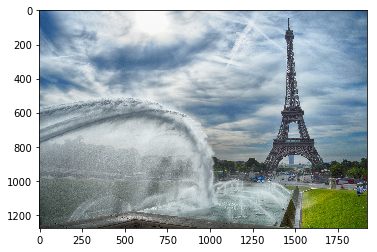What to remember from this assignment:

1. Preprocessing the dataset is important.
2. You implemented each function separately: initialize(), propagate(), optimize(). Then you built a model().
3. Tuning the learning rate (which is an example of a “hyperparameter”) can make a big difference to the algorithm. You will see more examples of this later in this course!

Finally, if you’d like, we invite you to try different things on this Notebook. Make sure you submit before trying anything. Once you submit, things you can play with include:
- Play with the learning rate and the number of iterations
- Try different initialization methods and compare the results
- Test other preprocessings (center the data, or divide each row by its standard deviation)

Bibliography:

• https://stats.stackexchange.com/questions/211436/why-do-we-normalize-images-by-subtracting-the-datasets-image-mean-and-not-the-c
SoftwareVersion
Python3.6.6 64bit [GCC 4.2.1 Compatible Apple LLVM 6.1.0 (clang-602.0.53)]
IPython7.0.1
OSDarwin 17.7.0 x86_64 i386 64bit
skimage0.14.1
numpy1.15.1
matplotlib3.0.0
h5py2.8.0
sklearn0.20.0
Mon Oct 15 08:33:43 2018 MDT